# How to AutoSum Multiple Rows and Columns in Excel?

Using the SUM method in Excel, we can normally total up the values in a column or row. In point of fact, rather than manually putting the SUM formula into a cell, we may use the AutoSum formula to calculate the sum of the values in a far more expedient manner. Within Excel, there is a formula known as AutoSum, which I shall explain below. Excel can automatically compute the total of a column or row of numbers when you ask it to do so.

Excel will automatically enter a formula to sum the numbers (one that makes use of the SUM function) whenever you click the AutoSum button.

## Look for the AutoSum Button

Step 1 − Simply choose a cell that is next to the values you want to add up, go to the Editing group on the Home tab of the spreadsheet, and click on the AutoSum button. After that, hit the Enter key to complete the process.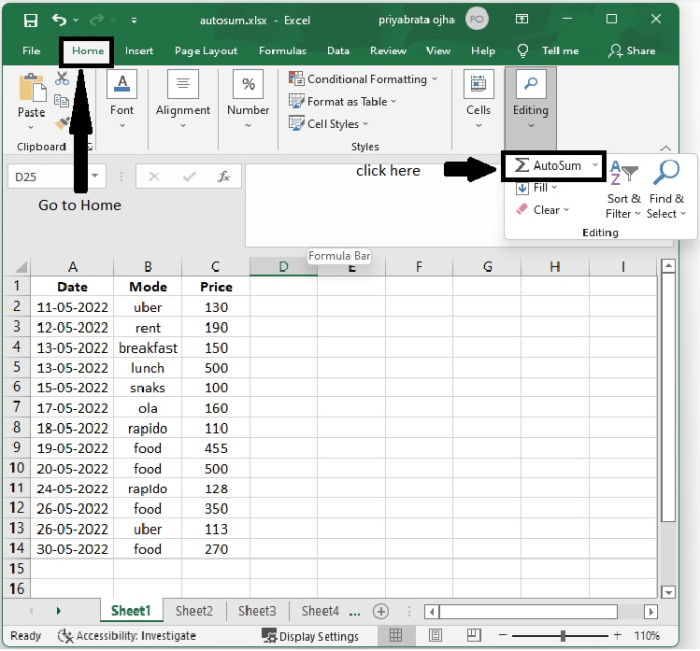Step 2 − You may calculate by going to the AutoSum menu and selecting Sum for AutoSum from the list of options there.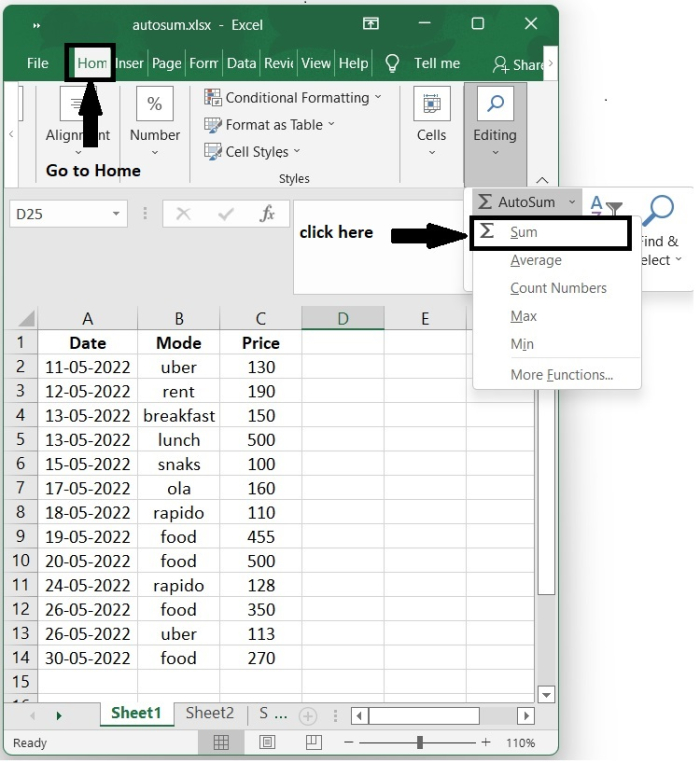And on the "Formulas" tab, the AutoSum button is located in the group labelled Function Library, where it is quite easy for us to locate it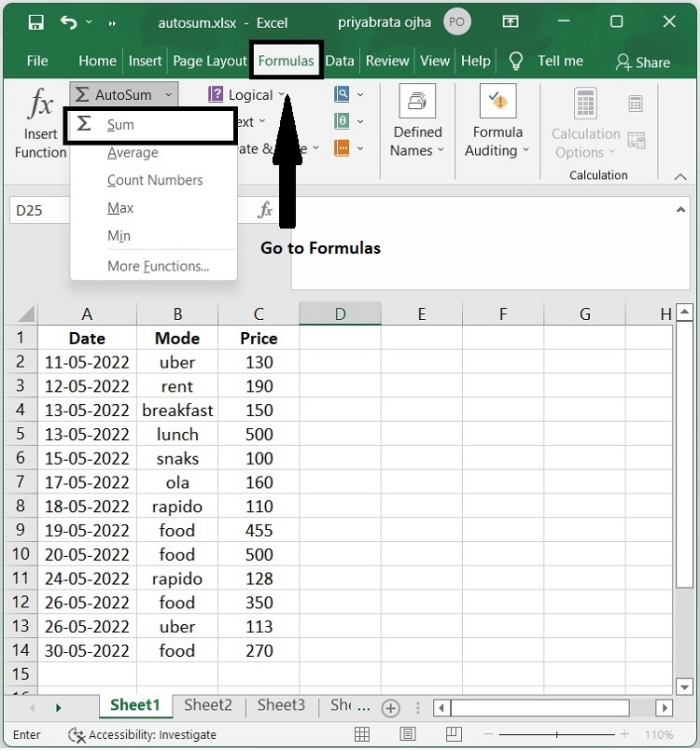## AutoSum Excel Single Columns

we rapidly get the total value for a list of data in Excel using the AutoSum function, but it can also assist us in calculating the sum values of individual rows and individual columns simultaneously.

Step 1 − Choose the individual column that you want to AutoSum, as seen in the screen picture below.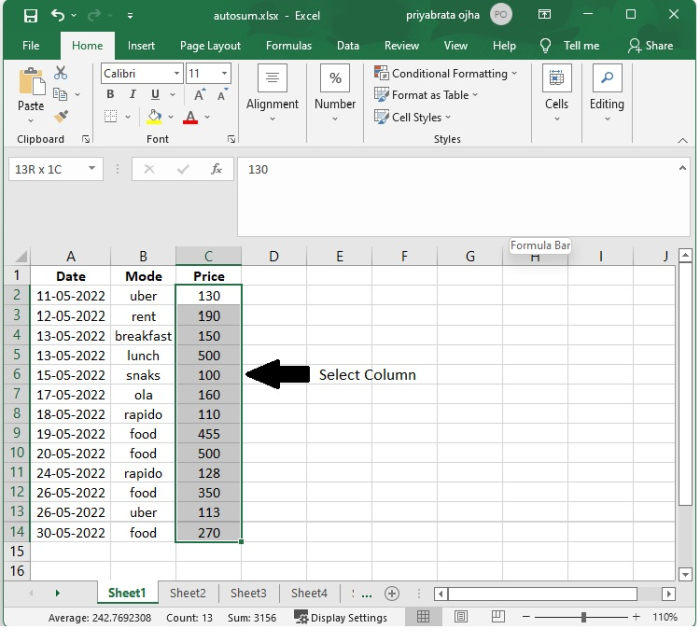Step 2 − You may also use the AutoSum feature by going to the Formula menu and selecting AutoSum from there, or by going to the Home menu and selecting AutoSum. And here is the result.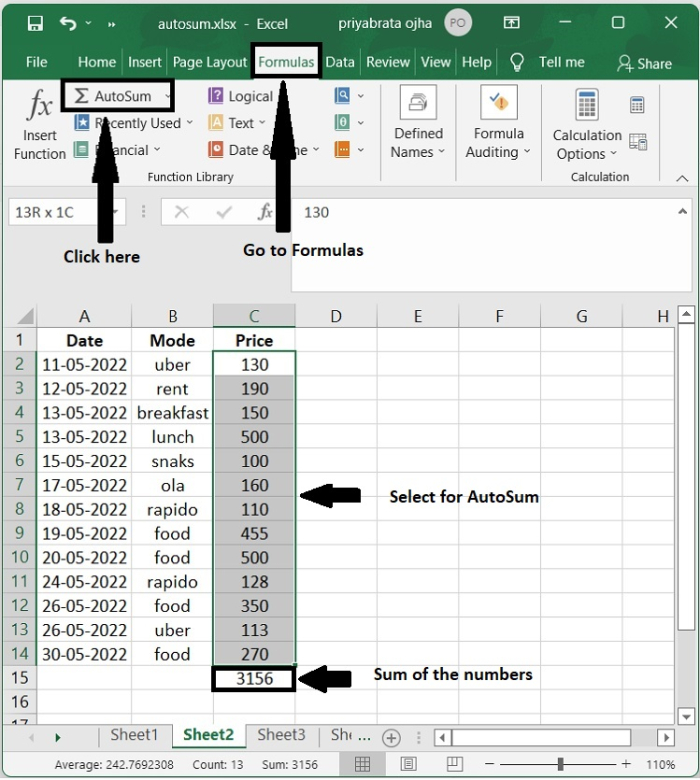## AutoSum Excel Single Rows

Step 1 − Choose the individual row that you want to AutoSum, as seen in the screen picture below.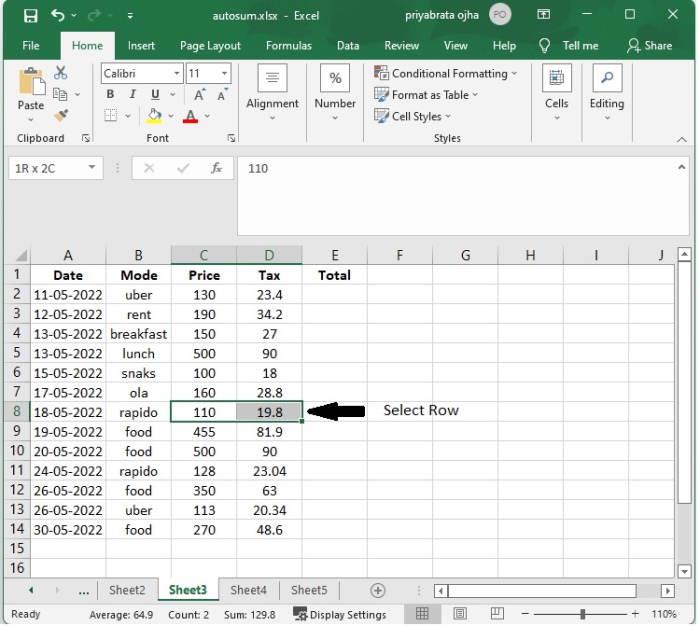Step 2 − You may also use the AutoSum feature by going to the Formula menu and selecting AutoSum from there, or by going to the Home menu and selecting AutoSum. And here is the result.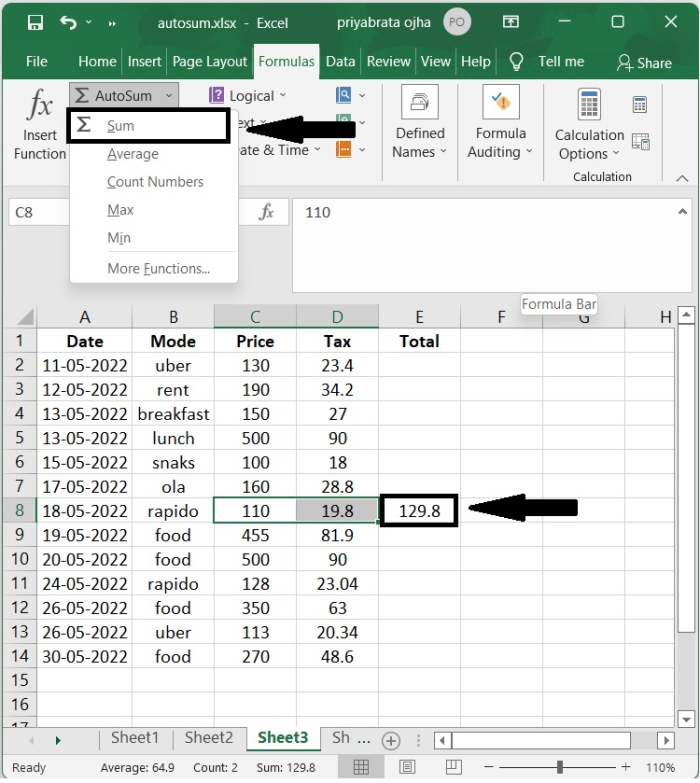## AutoSum Excel Multiple Rows/Columns

The AutoSum function in Excel is able to assist us in calculating the sum value for a list of data by calculating the sum values of multiple rows and multiple columns at the same time.

Step 1 − Select a number of rows and columns, together with the blank row and column that is directly to the right of these rows and below of columns, as shown in the following image.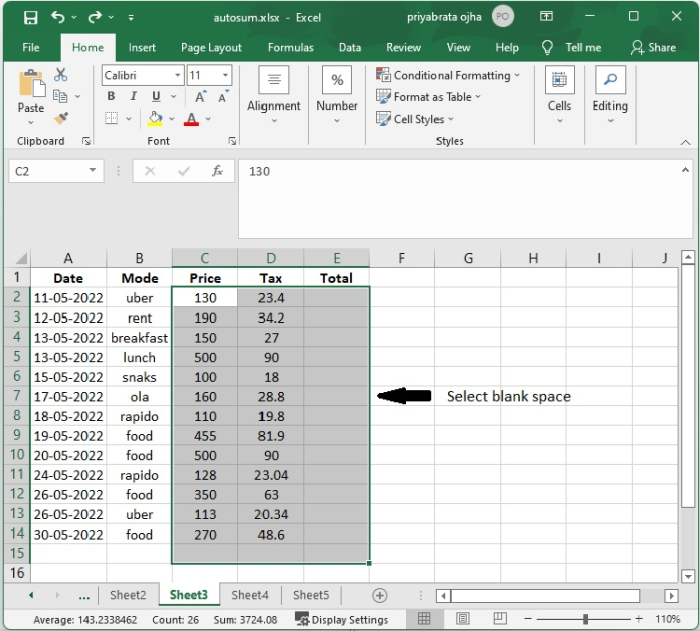Step 2 − You may also use the AutoSum feature by going to the Formula menu and selecting AutoSum from there, or by going to the Home menu and selecting AutoSum. And here is the result.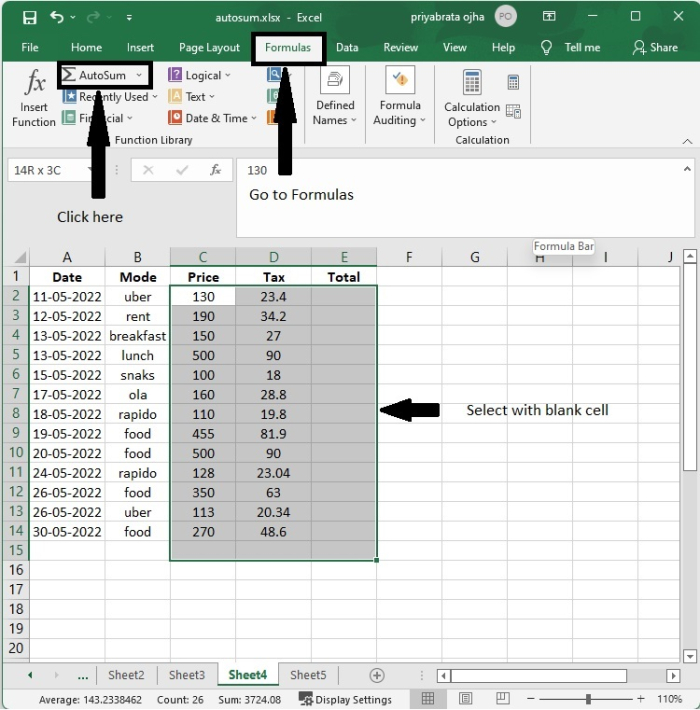Step 3 − Choose the range that you want the total to be for as well as the blank column right to this range and the blank row below this range and You may also use the AutoSum feature by going to the "Formula" menu and selecting "AutoSum" from there, or by going to the "Home" menu and selecting "AutoSum." And here is the result.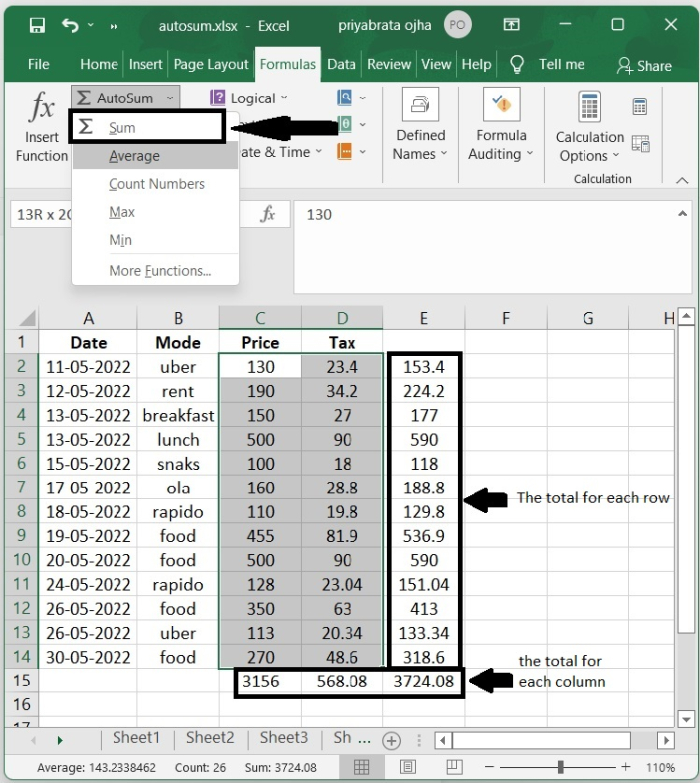## Conclusion

In this tutorial, you learnt how to automatically add the values of single rows and single columns. In addition, you also learnt ways to AutoSum the values of multiple rows and columns.